﻿ 基于DOD和PTD的北斗欺骗式干扰检测技术研究
«上一篇文章快速检索 高级检索

 应用科技2019, Vol. 46Issue (2): 35-41  DOI: 10.11991/yykj.2018070100

### 引用本文ZHANG Guoli, ZHANG Yao, TIAN Ye. Research of Beidou navigation satellite system spoofing detection based on DOD and PTD[J]. Applied Science and Technology, 2019, 46(2), 35-41. DOI: 10.11991/yykj.201807010.### 文章历史

1. 中国人民解放军92941部队，辽宁 葫芦岛 125000;
2. 哈尔滨工程大学 自动化学院，黑龙江 哈尔滨 150001

Research of Beidou navigation satellite system spoofing detection based on DOD and PTD
ZHANG Guoli1, ZHANG Yao2, TIAN Ye2
1. The People’s Liberation Army 92941 Troops, Huludao 125000, China;
2. College of Automation, Harbin Engineering University, Harbin 150001, China
Abstract: In order to detect spoofing attacks of Beidou navigation signals in the signal-tracking phase, we analyzed Beidou navigation signal system model in the tracking phase, then proposed the spoofing attacks algorithm based on power threshold detection (PTD) and Doppler offset detection (DOD), so as to realize spoofing attacks detection in the tracking phase by Beidou receiver. Simulations were implemented on the platform of Beidou software receiver, proving effectiveness of the proposed algorithm.
Keywords: Beidou satellite navigation system    spoofing attacks    Doppler shift    power threshold detection    carrier-to-noise ratio    detection probability    detection statistics    mobile variance

1 跟踪阶段系统模型分析

1.1 正常接收信号模型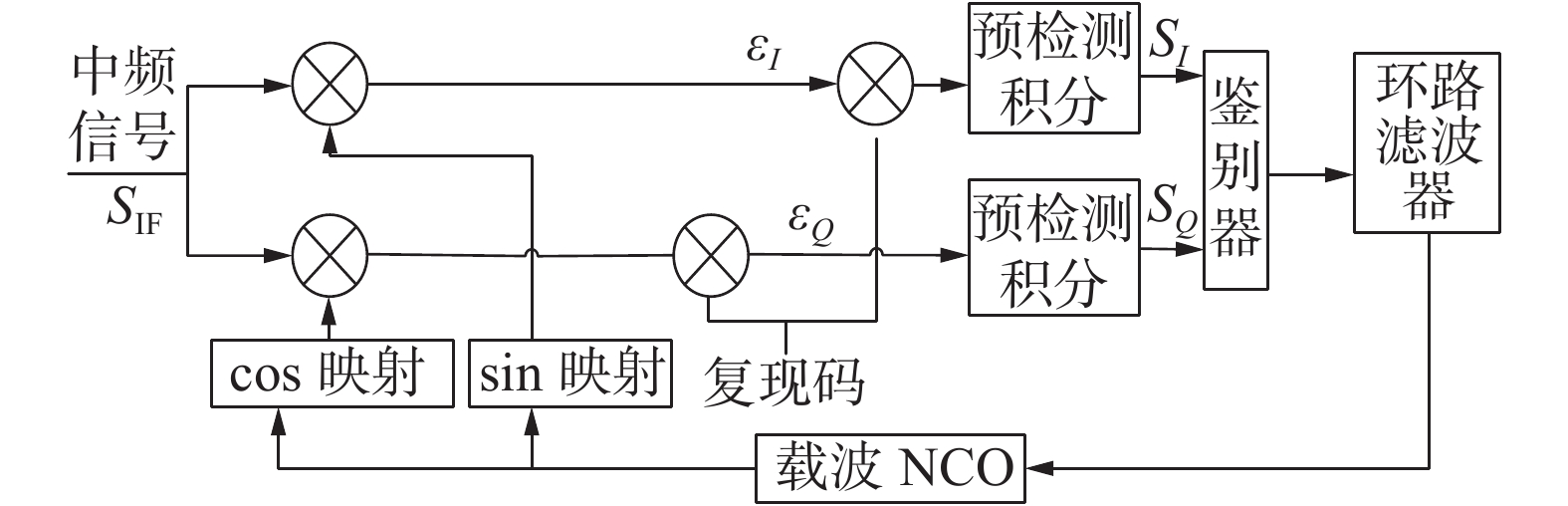Download: 图 1 接收机锁相环结构

 ${S_{{\rm{IF}}}}(t) = A(t)C(t)D(t)\sin ({\omega _{{\rm{IF}}}}t + {\theta _{{\rm{IF}}}}) + {n_{{\rm{IF}}}}$

 ${S_{{\rm{IF}}}}(t) = A(t)D(t){\rm{sin}}({\omega _{{\rm{IF}}}}t + {\theta _{{\rm{IF}}}}) + {n_{{\rm{IF}}}}$

 ${S_{{L_I}}}(t) = {\rm{sin}}({\omega _L}t + {\theta _L}){\kern 1pt} {\kern 1pt} {\kern 1pt}$
 ${S_{{L_Q}}}(t) = \cos ({\omega _L}t + {\theta _L}){\kern 1pt} {\kern 1pt}$

 \begin{aligned} \;\; {\varepsilon _I} = & A(t)D(t)\frac{{\cos \; {\varphi _1} - \cos {\varphi _2}}}{2} + {n_c}(t)\frac{{\sin \;{\varphi _1} + \sin \;{\varphi _2}}}{2} + \\ \;\; & {n_s}(t)\frac{{\cos \; {\varphi _1} - \cos \; {\varphi _2}}}{2} \end{aligned}
 \begin{aligned} \;\;\;\; {\varepsilon _Q} = & A(t)D(t)\frac{{\sin \; {\varphi _1} - \sin \; {\varphi _2}}}{2} + {n_c}(t)\frac{{\cos \; {\varphi _1} + \cos \; {\varphi _2}}}{2} + \\ \;\;\;\; & {n_s}(t)\frac{{\sin \; {\varphi _1} - \sin \; {\varphi _2}}}{2} \end{aligned}

${\varepsilon _I}$ ${\varepsilon _Q}$ 进行积分，积分时间的长度设置为 $T$ ，假设在积分时间内幅度和数据均不变，则积分后的信号为

 ${S_I}(t) = \frac{{ADT\cos \;{\varphi _1}}}{2} + \frac{{{N_c}\sin \; {\varphi _1}}}{2} + \frac{{{N_s}\cos \; {\varphi _1}}}{2}$
 ${S_Q}(t) = \frac{{ADT\sin \;{\varphi _1}}}{2} + \frac{{{N_c}\cos \; {\varphi _1}}}{2} + \frac{{{N_s}\sin \; {\varphi _1}}}{2}$

1.2 欺骗信号入侵后的接收信号模型

 ${S_S}(t) = {A_S}(t){C_S}(t){D_S}(t){\rm sin}({\omega _S}t + {\theta _S})$

 $S(t) = {S_{{\rm{IF}}}}(t) + {S_S}(t)$

 $S(t) = C(t)D(t){A_M}(t){\rm cos}({\omega _{IF}}t + {\theta _M}) + n(t)$

2 跟踪阶段欺骗检测方法设计

2.1 信号功率异常检测算法设计

 \begin{aligned} \sigma _{MV}^2 =& \frac{1}{W}\sum\nolimits_{k = n - W + 1}^n {{{[x(k) - \overline {x(n)} ]}^2}} - \overline {x(n)} = \\ & \overline {{x^2}(n)} - \overline {x(n)} \end{aligned}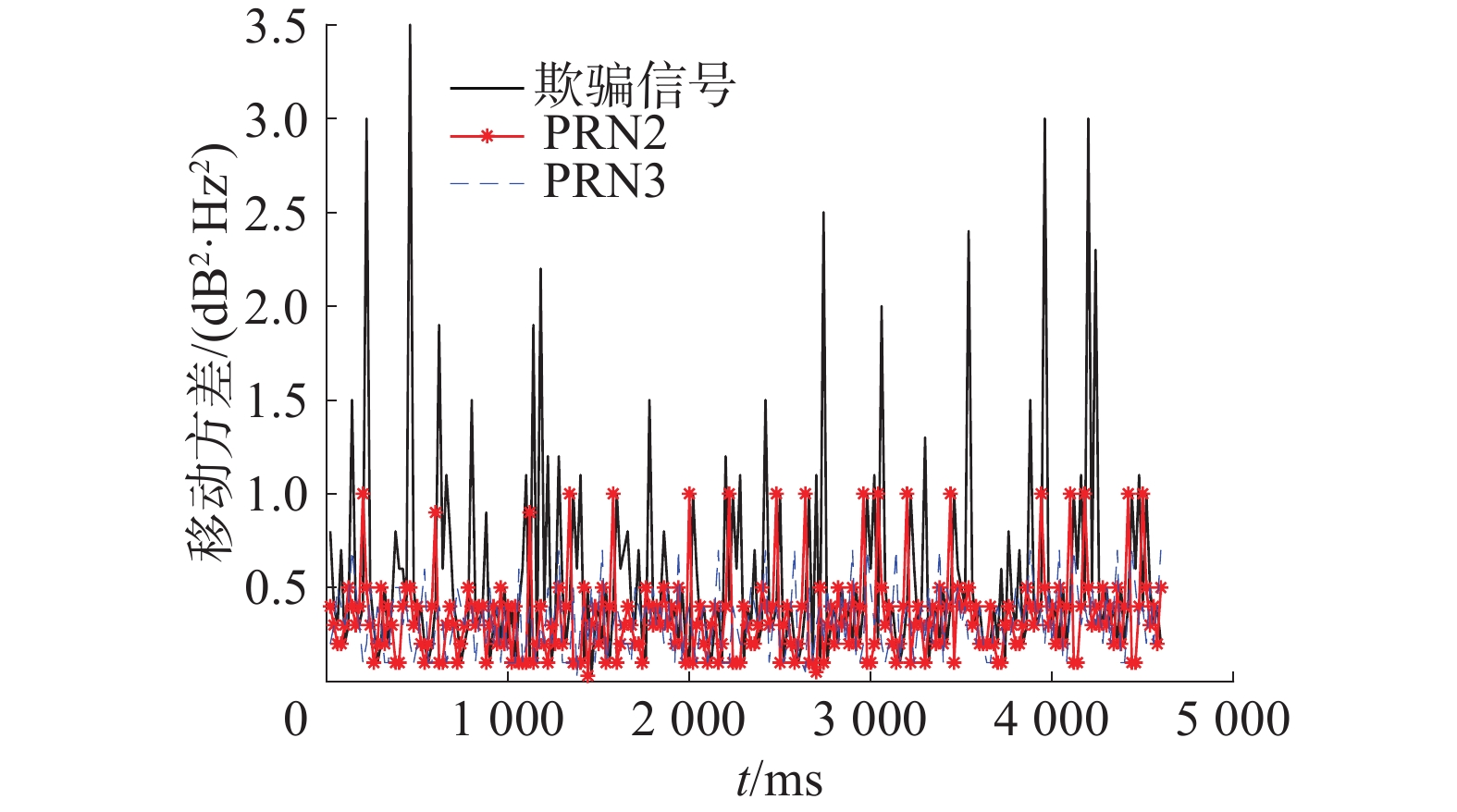Download: 图 2 载噪比移动方差
2.2 多普勒频移异常检测算法设计

 ${f_r} = {f_c}(1 - \frac{{a{v_r}}}{c})$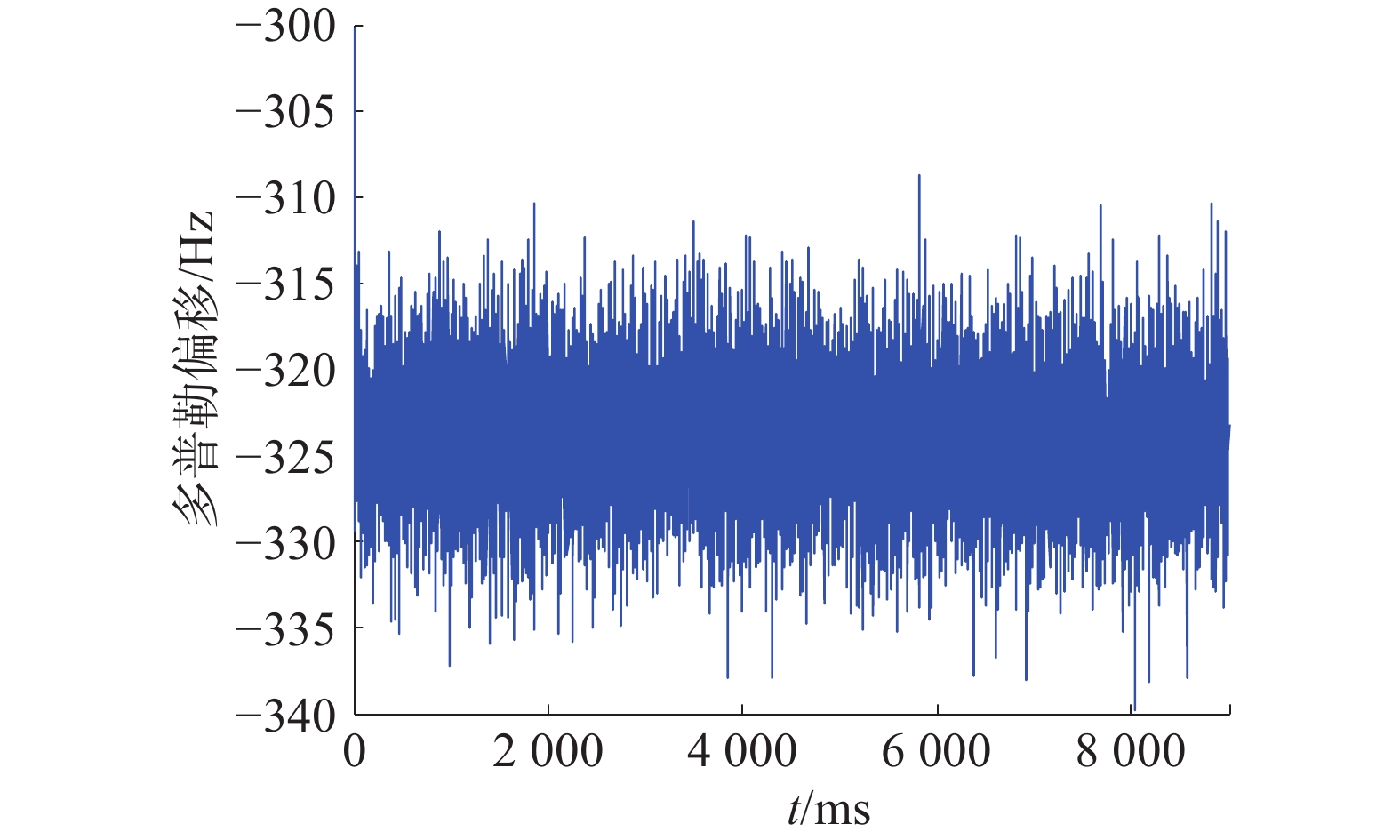Download: 图 3 跟踪阶段卫星多普勒频移量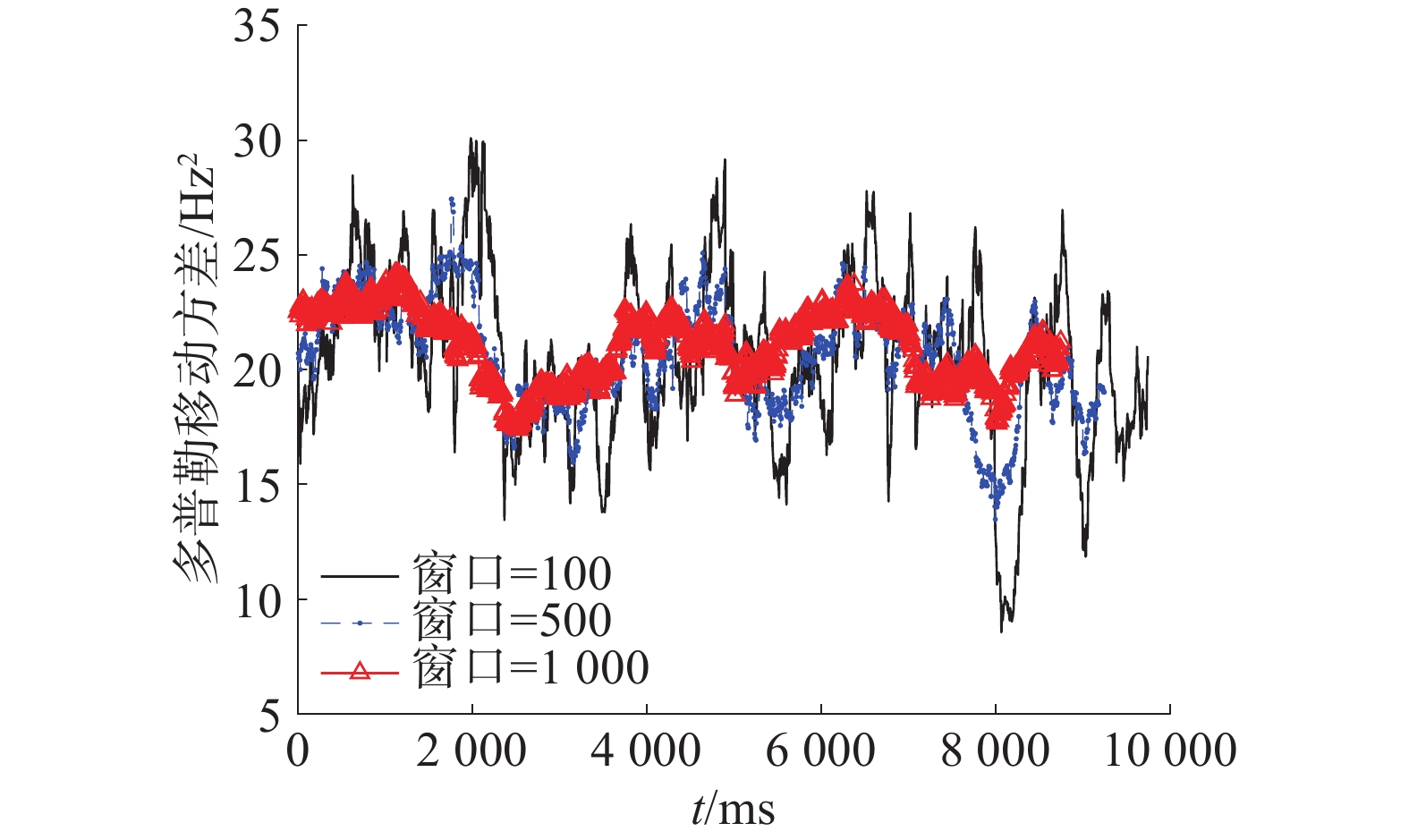Download: 图 4 跟踪阶段不同窗口大小下卫星多普勒频移量移动方差
3 仿真实验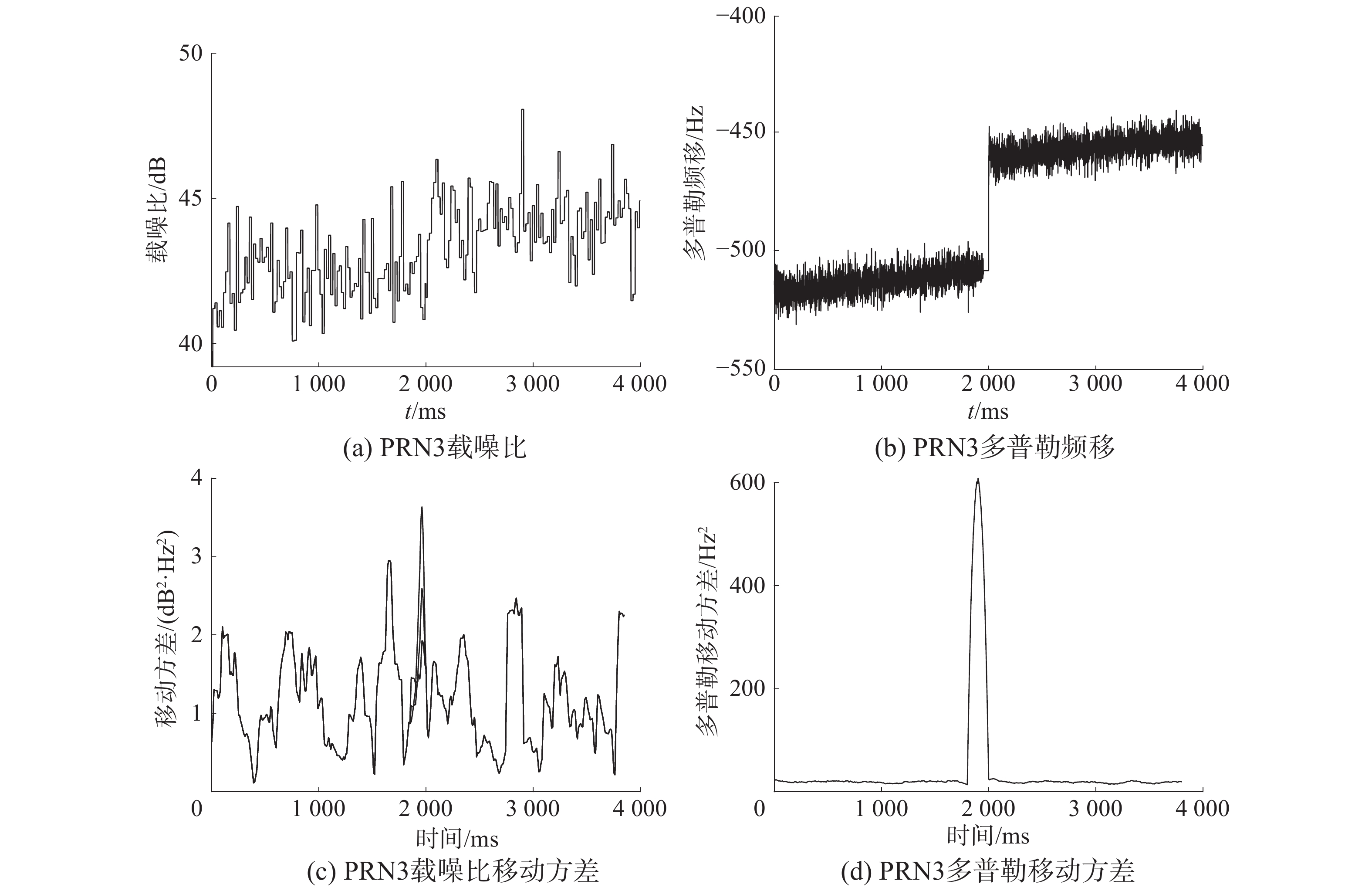Download: 图 5 产生式欺骗干扰下仿真图 1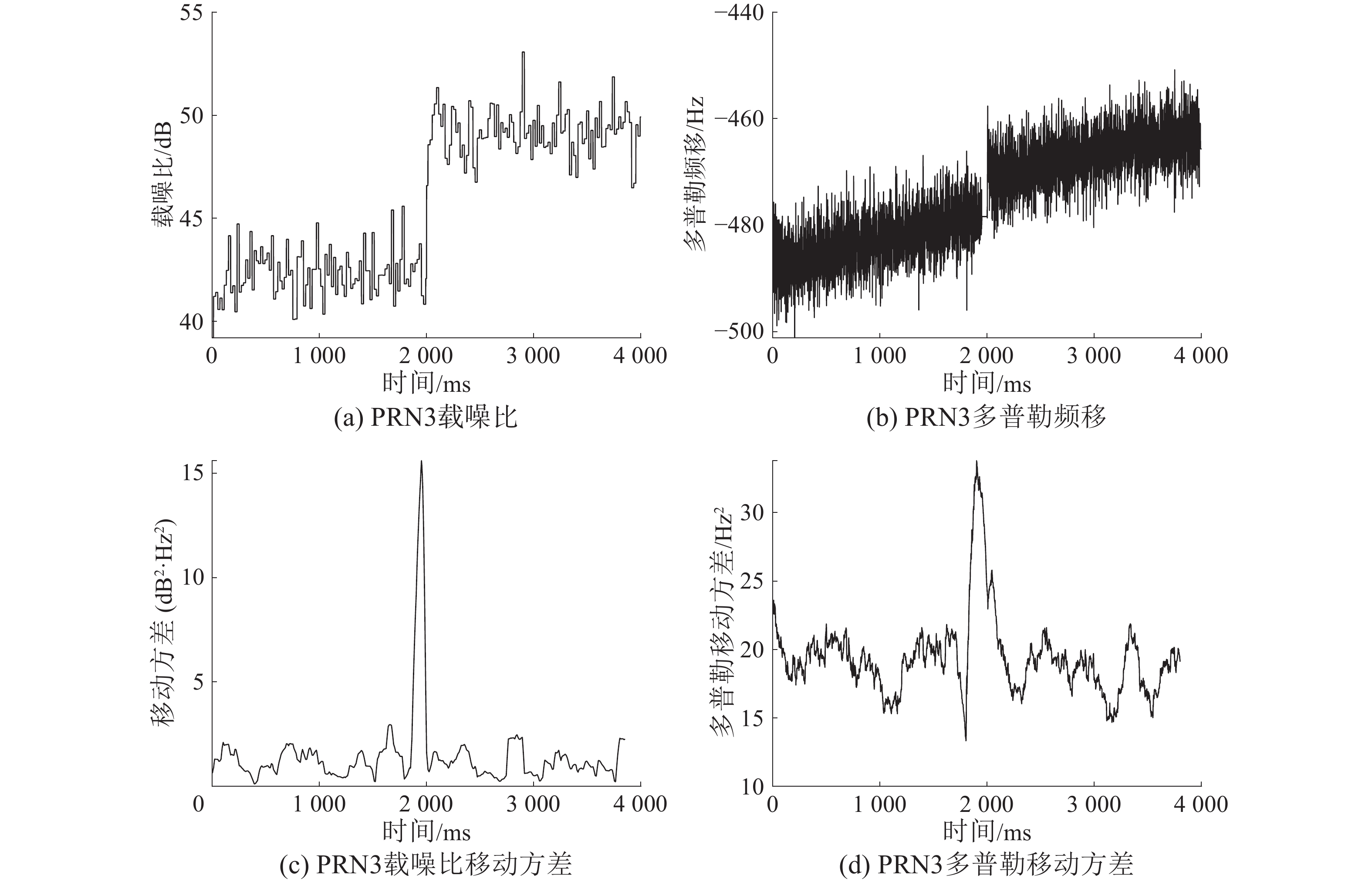Download: 图 6 产生式欺骗干扰下仿真图 2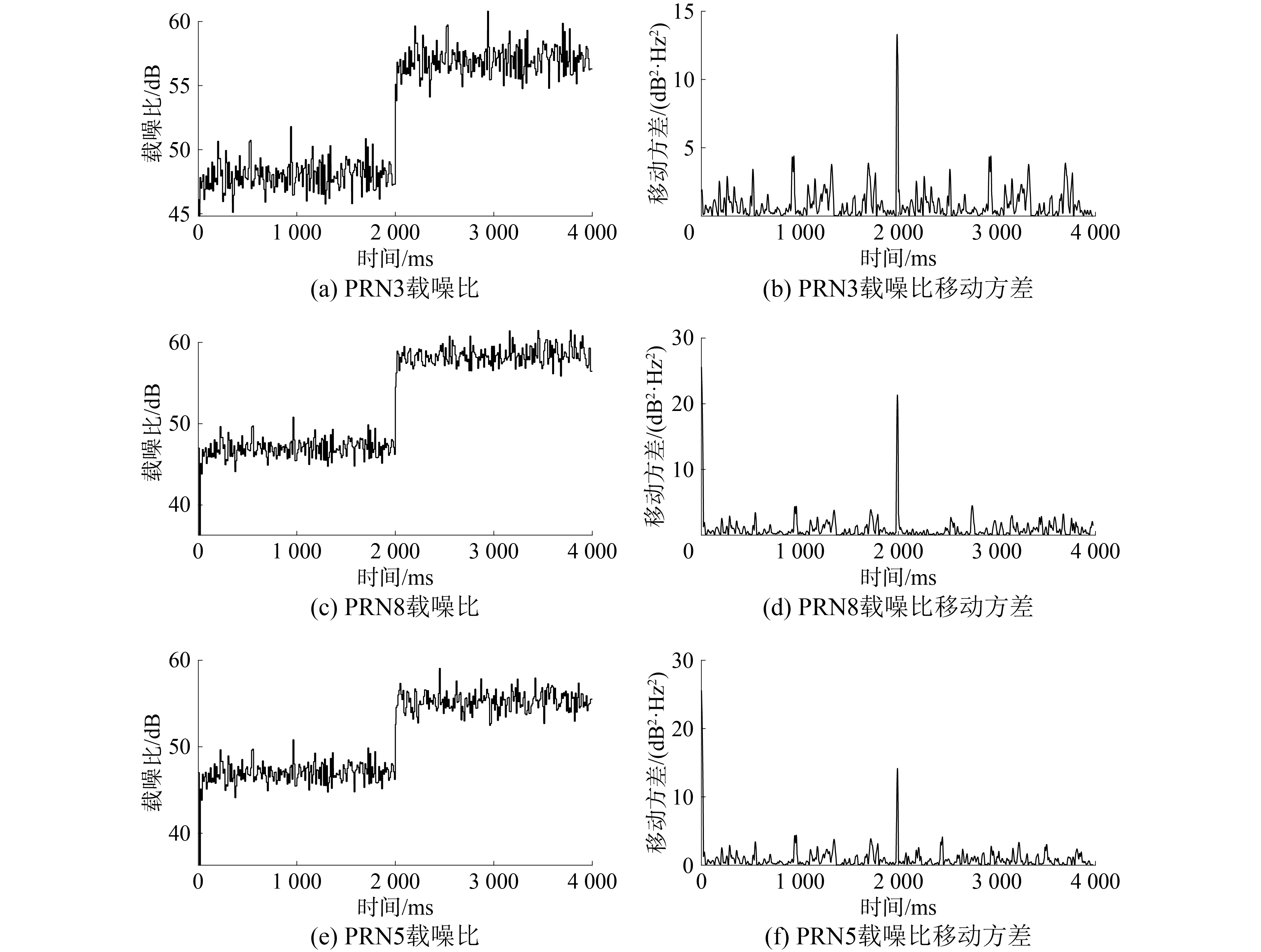Download: 图 7 转发式欺骗干扰功率异常检测仿真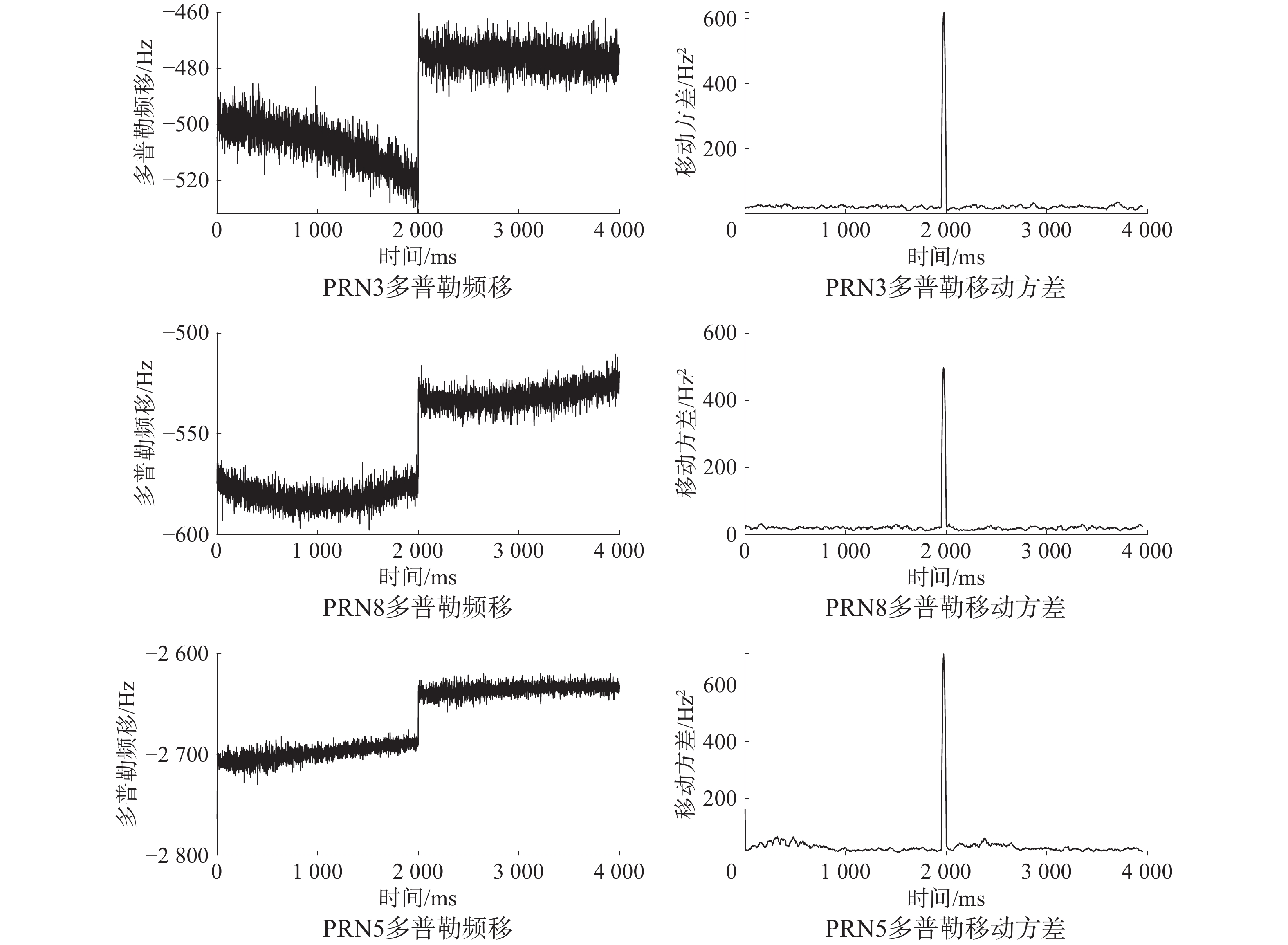Download: 图 8 转发式欺骗干扰多普勒频移检测仿真

 ${p_{\rm df}}(x) = \frac{1}{{x\sigma \sqrt {2{\rm{{\text{π} }}}} }}\exp ( - \left( {\log (x) - \mu } \right)/2{\sigma ^2})$
 $c_{\rm df}(x) = \frac{1}{2} + \frac{1}{2}{\rm erf}\left[ {\frac{{\ln (x) - \mu }}{{\sqrt {2{\sigma ^2}} }}} \right]$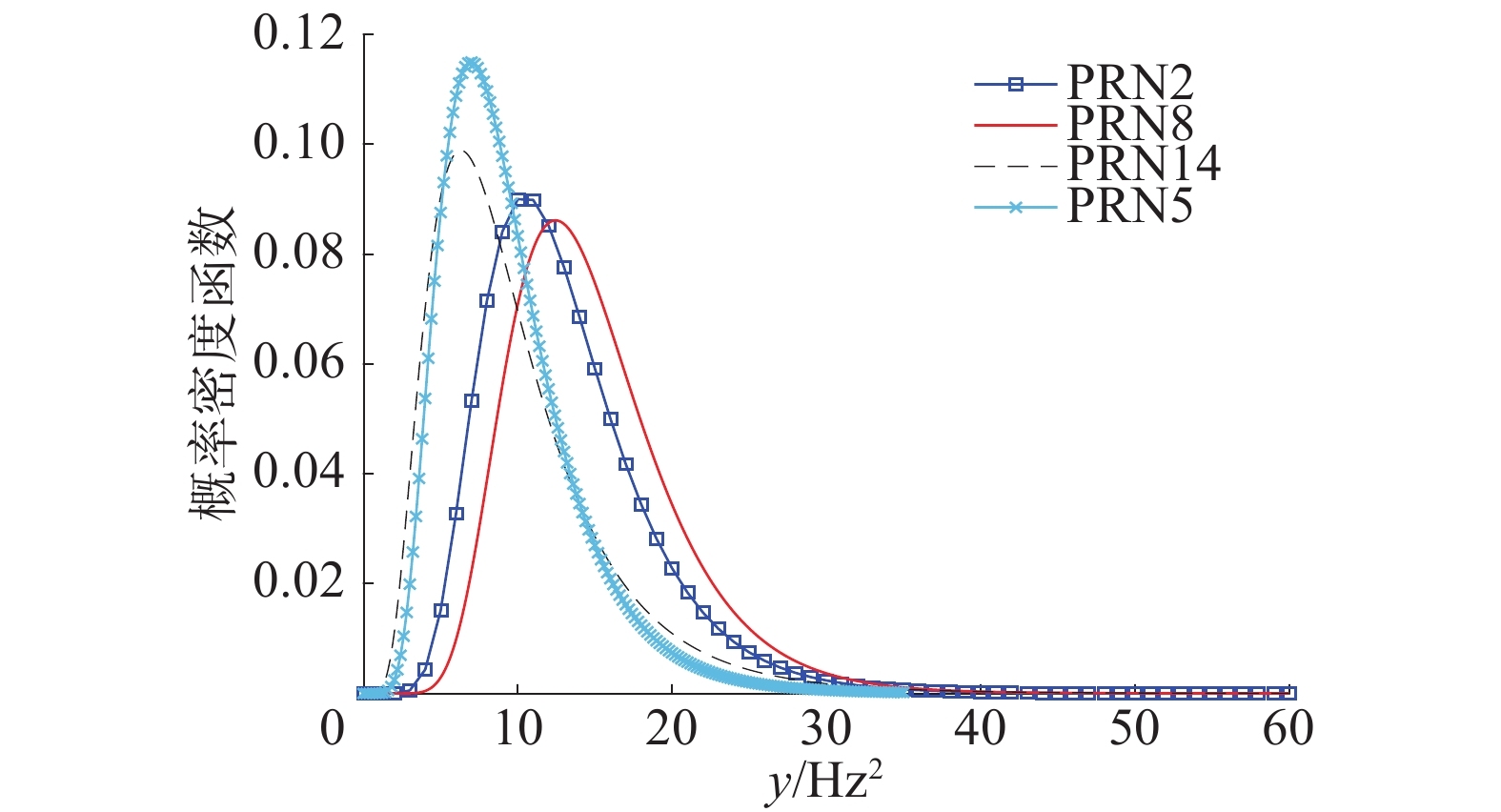Download: 图 9 多普勒频移检测概率密度函数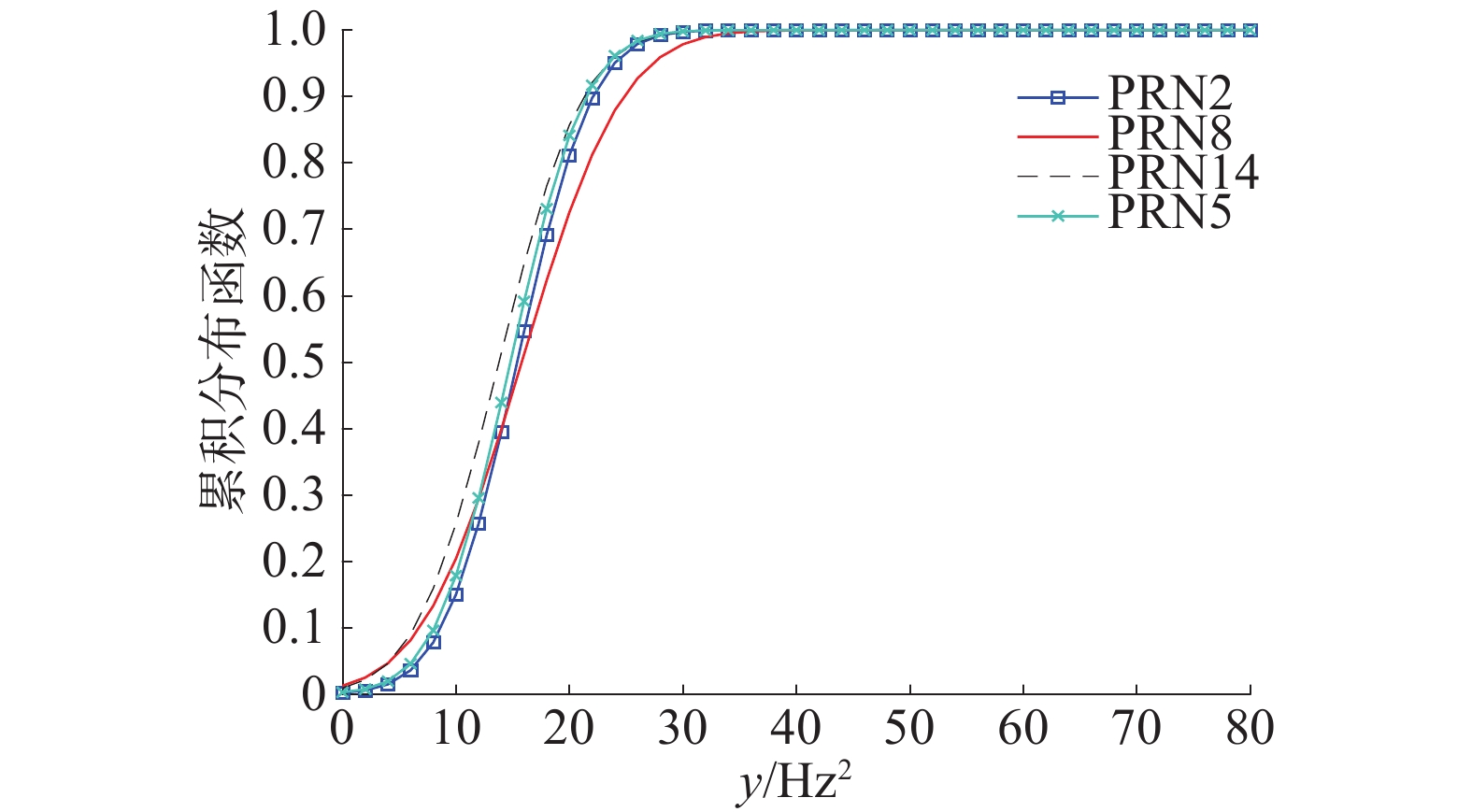Download: 图 10 多普勒频移检测积累分布函数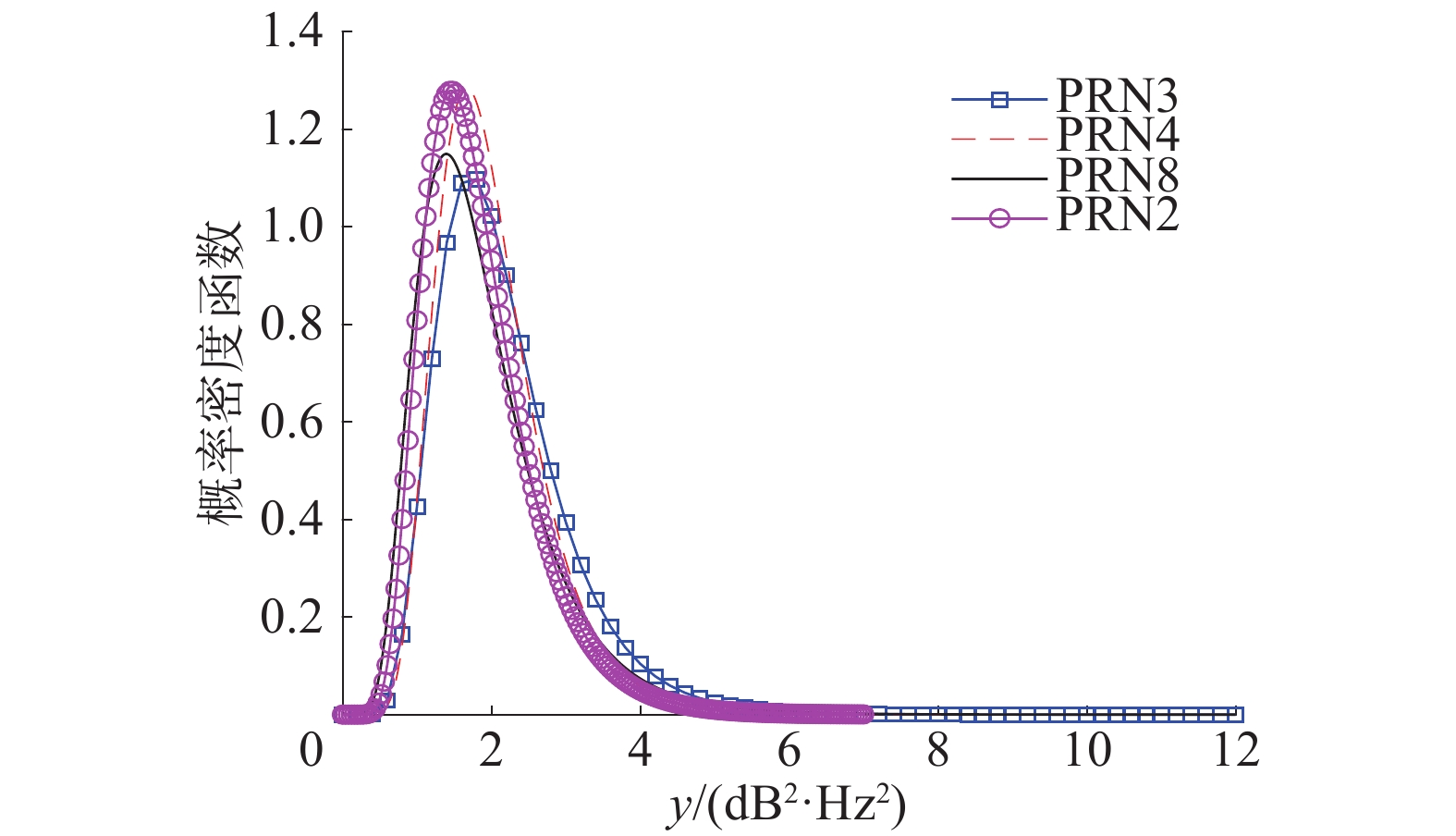Download: 图 11 功率异常检测概率密度函数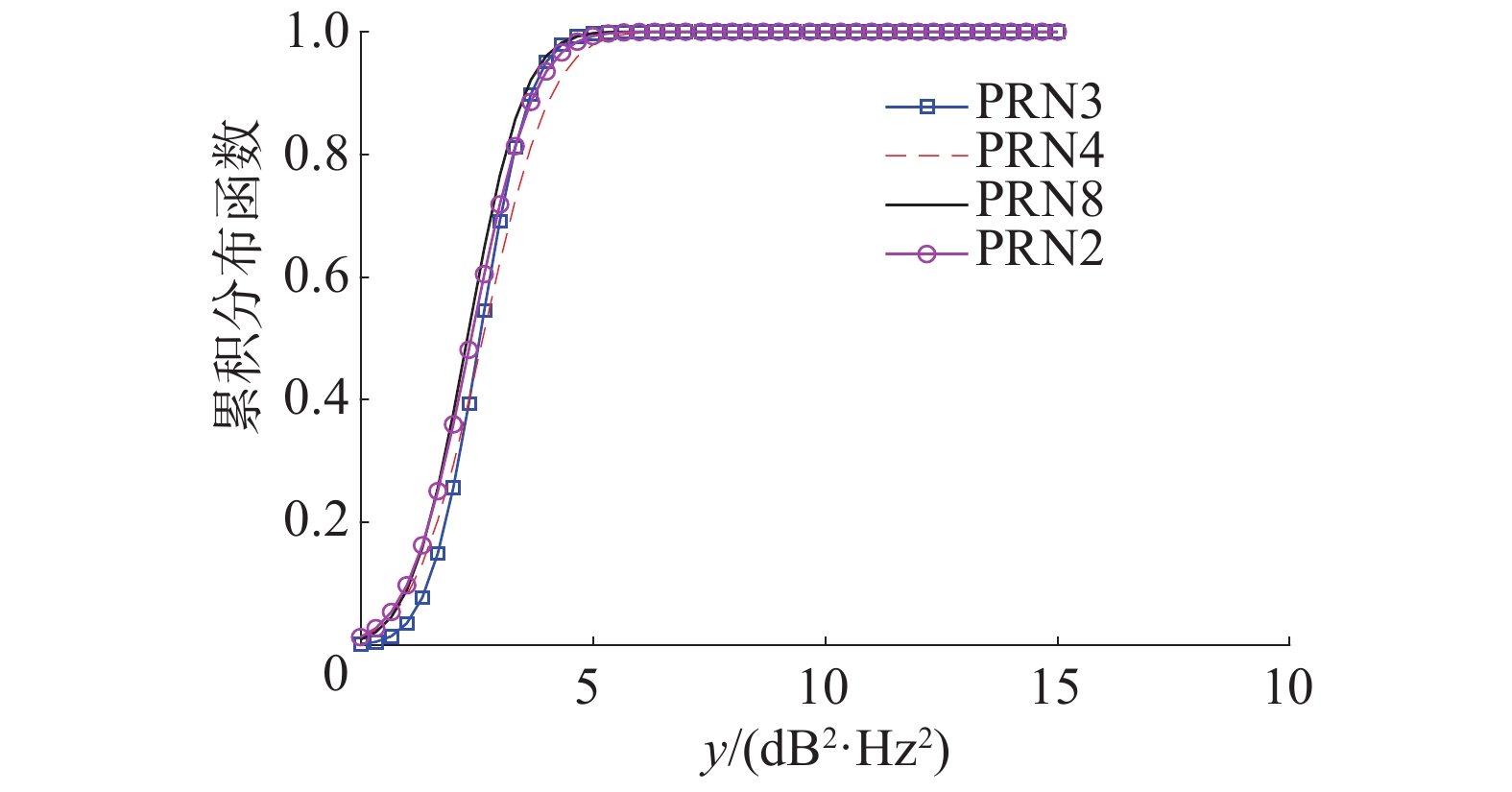Download: 图 12 功率异常检测积累分布函数

 ${P_{\rm fa}} = P_{\rm fa}^{\rm PTD} \cdot P_{\rm fa}^{\rm DOD} = 2.271\;3 \times {10^{ - 8}}$

4 结束语

  何四华, 李天伟, 韩云东. 导航战中GPS干扰技术的研究[J]. 舰船电子对抗, 2004, 27(1): 24-27. DOI:10.3969/j.issn.1673-9167.2004.01.006 (0)  王茂锋, 王少华. GNSS抗欺骗干扰技术解析[J]. 通讯世界, 2015(13): 56-57. DOI:10.3969/j.issn.1006-4222.2015.13.039 (0)  谢红. 对GPS转发式干扰技术研究[J]. 无线电工程, 2008, 38(3): 34-36. DOI:10.3969/j.issn.1003-3106.2008.03.012 (0)  PSIAKI M L, O’HANLON B W, BHATTI J A, et al. GPS spoofing detection via dual-receiver correlation of military signals[J]. IEEE transactions on aerospace and electronic systems, 2013, 49(4): 2250-2267. DOI:10.1109/TAES.2013.6621814 (0)  黄龙, 龚航, 朱祥维, 等. 针对GNSS授时接收机的转发式欺骗干扰技术研究[J]. 国防科技大学学报, 2013, 35(4): 93-96. DOI:10.3969/j.issn.1001-2486.2013.04.017 (0)  黄龙, 吕志成, 王飞雪. 针对卫星导航接收机的欺骗干扰研究[J]. 宇航学报, 2012, 33(7): 884-890. DOI:10.3873/j.issn.1000-1328.2012.07.005 (0)  谢钢. GPS原理与接收机设计[M]. 北京: 电子工业出版社, 2009: 30-32. (0)  赵琳, 丁继成, 马雪飞. 卫星导航原理及应用[M]. 西安: 西北工业大学出版社, 2011: 88-91. (0)  王伟, 陶业荣, 王国玉, 等. GPS欺骗干扰原理研究与建模仿真[J]. 火力与指挥控制, 2009, 34(6): 115-118. DOI:10.3969/j.issn.1002-0640.2009.06.032 (0)  周坤芳, 周湘蓉, 余军浩. GPS接收机码/载波跟踪环技术及其抗干扰能力分析[J]. 电子信息对抗技术, 2006, 21(2): 36-39. DOI:10.3969/j.issn.1674-2230.2006.02.009 (0)  曹可劲, 彭煊困, 李豹, 等. 基于信噪比测量的欺骗干扰检测方法[J]. 计算机测量与控制, 2016, 24(4): 29-32, 35. (0)  王雪冬, 吴盘龙, 恽鹏. 基于Doppler估计的弹载接收机抗欺骗干扰研究[J]. 导航与控制, 2017, 16(5): 7-12. DOI:10.3969/j.issn.1674-5558.2017.05.002 (0)